# GED Math : Median

## Example Questions

← Previous 1 3 4

### Example Question #1 : Median

Find the median of the following set of numbers: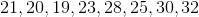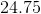This set of numbers does not have a median.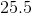Explanation:

The median of a set of numbers is the number that is in the middle of the set when the numbers are written in increasing order (from smallest to largest).

When a list of numbers includes an even quantity, like the list in this question, you need to find the mean (average) of the two numbers in the middle.

The steps to find the median of this number set are below:

1. Rewrite the list of numbers so that the numbers are written in increasing order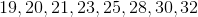2. Identify the number(s) in the middle of the set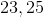3. Since there are two numbers in the middle of the set, find the average of the two numbers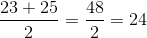### Example Question #1 : Median

Given the data set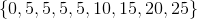, which of the following quantities are equal to each other/one another?

I: The mean

II: The median

III: The mode

II and III only

I,  II, and III

I and III only

I and II only

II and III only

Explanation:

The median of a data set with an odd number of elements is the middle value when the set is arranged in ascending order; the middle value of this nine-element set is the fifth value, 5.

The mode of a data set is the most frequently occurring element. Here, only 5 appears multiple times, so it is the mode.

The mean of a data set is the sum of its elements divided by the number of elements, which here is 9: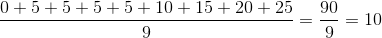The mean and the mode are equal, but the mean is different. The correct answer is "II and III only".

### Example Question #3 : Median

Which of the following elements can be added to the data set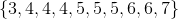so that its median remains unchanged?

I:II:III:I and III only

I and II only

II and III only

I, II, and III

I, II, and III

Explanation:

The median of a data set with an even number of elements is the mean of the two elements that are in the middle when the elements are arranged in ascending order, such as this one. The middle elements of this ten-element set are both 5, so this is the median.

The median of a data set with an odd number of elements is the element that is in the middle when the elements are arranged in ascending order.

If 0 is added to the set, it becomes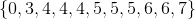.

If 5 is added, it becomes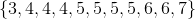.

If 100 is added, it becomes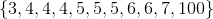.

In all cases, the middle element is 5, so the median remains 5. The correct choice is "I, II, and III".

### Example Question #4 : Median

Veronica went to the mall and bought several gifts for her sister's birthday. The prices of the items were the following:

$8,$10, $6,$7, $10,$2, $5,$3, $4 What is the median price of the gifts? Possible Answers:$4.50

$8$2

$10$6

$6 Explanation: To find the median, first reorder the prices in ascending order:$2,  $3,$4, $5,$6, $7,$8, $10,$10

The median is the middle numbein a data set.

Here, the middle number in this set of nine prices is the 5th number, which is $6.$2,  $3,$4, $5,$6, $7,$8, $10,$10

### Example Question #5 : Median

Philip went to a family reunion this weekend and met his extended cousins, whose ages were the following:

10, 21, 7, 8, 19, 20, 8, 15, 17, and 13.

What is the median age?

17

13

10

14

8

14

Explanation:

The median is the middle number in a data set.

To find the median, rearrange the numbers in ascending order and find the middle number:

Given: 10, 21, 7, 8, 19, 20, 8, 15, 17, 13.

In order: 7, 8, 8, 10, 13, 15, 17, 19, 20, 21.

Since the data set has 10 numbers, which is even, the median is the average of the two middle numbers. The two numbers in the middle of this data set are 13 and 15. Their average, or the number in between them, is 14.

Therefore the median age is 14.

### Example Question #1921 : Ged Math

Use the following data set of test scores to answer the question: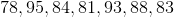Find the median.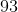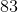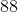Explanation:

To find the median score, we will first arrange the numbers in ascending order. Then, we will find the number in the middle of the set.

So, given the setwe will arrange the numbers in ascending order (from smallest to largest). So, we get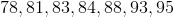Now, we will find the number in the middle.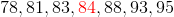We can see that it is 84.

Therefore, the median score of the data set is 84.

### Example Question #1922 : Ged Math

A Science class took an exam. Here are the scores of 9 students: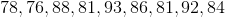Find the median score.Explanation:

To find the median score, we will first arrange the scores in ascending order. Then, we will find the score in the middle of the set.

So, given the setwe will first arrange them in ascending order (from smallest to largest). So, we get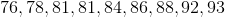Now, we will find the score in the middle of the set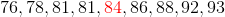we can see that it is 84.

Therefore, the median score is 84.

### Example Question #1923 : Ged Math

A class took a Math exam.  Here are the test scores of 11 students.Find the median.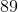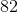Explanation:

To find the median of a data set, we will first arrange the numbers in ascending order. Then, we will find the number in the middle of the set. So, given the set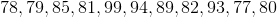we will arrange the numbers in ascending order (from smallest to largest). We get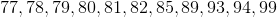Now, we will find the number in the middle of the set.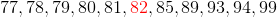Therefore, the median of the data set is 82.

### Example Question #1924 : Ged Math

Solve for the median: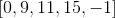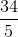Explanation:

Reorder the numbers from least to greatest.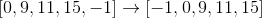In an odd set of data, the median is the central number of this data set.

The answer is:### Example Question #1925 : Ged Math

Identify the median: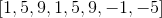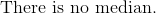Explanation:

Reorder all the numbers in chronological order.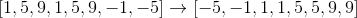The median of an even set of numbers is the average of the central two numbers.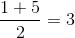The median is:← Previous 1 3 4